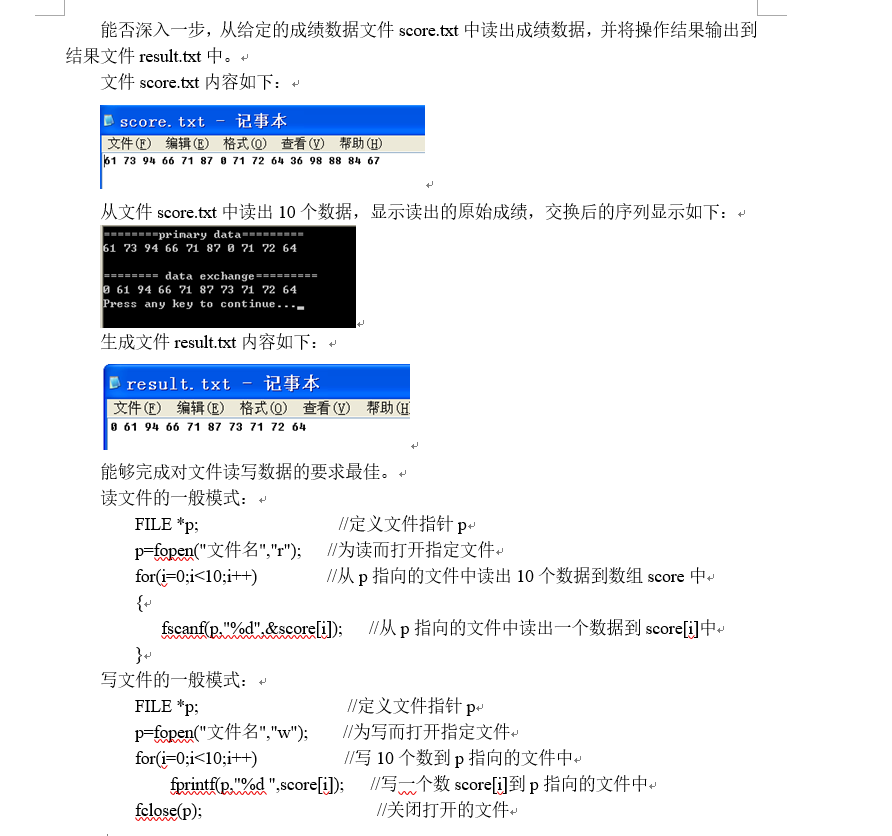3个回答

1.若最小数据有重复的，那么是应该吧所有的最小数据都调到前面，还是只移动一个，还是把所有的最下数据都去掉然后在前面只添加一个最小数据？
2.除最小数据外，其他数据 的位置是否能换更换

``````#include<stdio.h>
#include<malloc.h>
void find(int* p, int* min1, int* min2){  //注意此处若有相同的最小值或者次小值数据，只会将其中一个调整顺序
int cnt1, cnt2;
cnt1 = cnt2 = (*p);
(*min1) = (*min2) = 0;
for (int j = 0; j != 10; j++){
if (*(p + j) < cnt1) {
*min1 = j; cnt1 = *(p + j);
}
else if (*(p + j) < cnt2){
(*min2) = j; cnt2 = *(p + j);
}
}
}
int main()
{
FILE*fp;
fopen_s(&fp, "data.txt", "r");
printf("primary data\n");
int*p = (int*)malloc(sizeof(int)* 10);
for (int i = 0; i != 10;i++){
fscanf_s(fp, "%d", p+i);
printf("%d ",*(p+i));
}
int min1, min2;
int size = 10;
find(p, &min1, &min2);
printf("\ndata exchange\n");
printf("%d,%d\n", min1, min2);
FILE* fp_w;
fopen_s(&fp_w, "result.txt", "w");
if (min1 == min2){
printf("%d ", *(p + min1)); fprintf(fp_w, "%d ", *(p + min1));
}
else {
printf("%d %d ", *(p+ min1), *(p + min2)); fprintf(fp_w, "%d ", *(p + min1));
fprintf(fp_w, "%d ", *(p + min2));
}
for (int j = 0; j < size; j++)
{
if (j == min1 || j == min2)continue;
printf("%d ", *(p + j));
fprintf(fp_w, "%d ", *(p + j));
}
fclose(fp_w);
//free(q);   此处不能释放空间，因为当程序还没有结束，即在free到return 之间，程序将向目标文件写入数据，释放数组会造成错误
return 0;
}
``````HannanKan 中间有一条测试语句，楼主自己删吧“printf（“%d %d”，min1，min2）；”
3 年多之前 回复

#include
#include

using namespace std;

int main()
{
int score;
int s,i,j,k,m;
ifstream infile;
infile.open("C:\score.txt ");
if(!infile)
{
cout<<"error:unable to open input file:" < return -1;
}
i=0;
while(infile>>s)
score[i++]=s;

``````for(i=0;i<10;i++)
cout<<score[i]<<" ";

cout<<endl;
infile.close();
infile.clear();

m=10;
for(i=0;i<10;i++){
m--;
for(j=0;j<m;j++)
{
if(score[j]>score[j+1])
{
k=score[j];
score[j]=score[j+1];
score[j+1]=k;
}
}
}

for(i=0;i<10;i++)
cout<<score[i]<<" ";

ofstream outfile;
outfile.open("C:\\result.txt ");
if(!outfile)
{
cout<<"error:unable to open input file:" <<endl;
return -1;
}
i=0;
do
{
outfile<<score[i++]<<" ";
}while(i<10);

outfile.close();

return 0;
``````

}

``````
``````